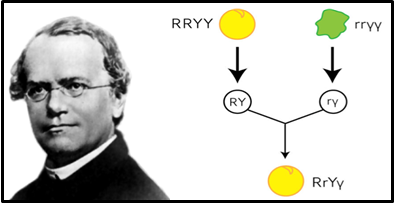https://blogs.biomedcentral.com/bmcseriesblog/2015/03/08/celebrating-150-years-mendelian-genetics/

# Mendelian Inheritance: Part III

If you have read the first and second part you may have understood that this is our third and final part. In this part we are going to discuss

• Monohybrid, dihybrid and trihybrid inheritance,
• Back cross and test cross and
• Probability in Mendelian inheritance, Chi-square test.  So let’s not waste any more time.

## Monohybrid, Dihybrid and Trihybrid Inheritance

KEY CONCEPT

Genes encode proteins that produce a diverse range of traits.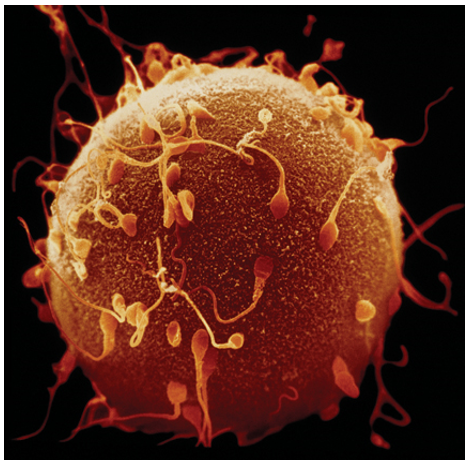Traits, Genes and Alleles

The same gene can have many versions called alleles.

Get Free Netflix Now

Best safe and secure cloud storage with password protection

Best Money Earning Website 100\$ Day

Best ever Chat Forum

#1 Top ranking article submission website

• A gene is a piece of DNA that directs a cell to make a certain protein.
• Each gene has a locus, or a specific position on a pair of homologous chromosomes.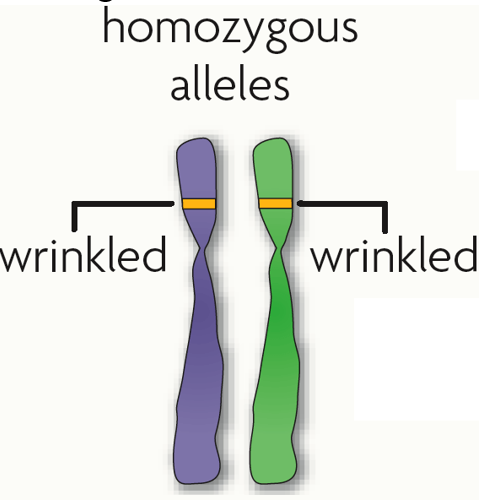• An allele is any alternative form of a gene occurring at a specific locus on a chromosome. Alleles are often represented by letters.
 Each parent donates one allele for every gene. Homozygous describes two alleles that are the same at a specific locus. Heterozygous describes two alleles that are different at a specific locus.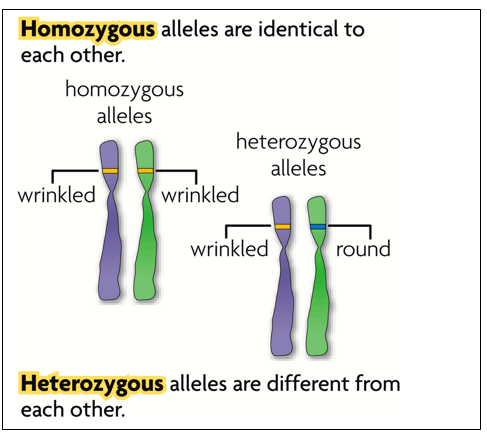Genes influence the development of traits.

All of an organism’s genetic material is called the genome.• A genotype refers to the makeup of a specific set of genes. Examples:  Tt, RR, bb
• A phenotype is the physical expression or appearance of a trait. Examples:  tall, round, blue
• Alleles are often represented using letters.
 Dominant alleles are represented by uppercase letters; recessive alleles by lowercase letters. A dominant allele is expressed as a phenotype when at least one allele is dominant. (Rr or RR = dominant; therefore the pea is round) A recessive allele is expressed as a phenotype only when two copies are present. (rr = recessive; therefore the pea is wrinkled)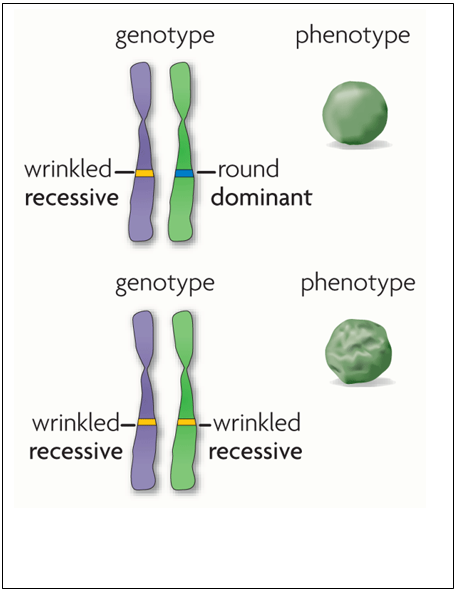These are few questions that might come in your mind:

• Mendel’s experiment and results
• Reason of his success
• How the traits are inherited???
• Outcomes of Mendel’s experiment
• Principles of segregation
• Monohybrid and dihybrid cross

Monohybrid Cross

• A monohybrid is a hybrid in which the parents differ in only one trait of interest. (They may differ in other traits as well, but the other differences are ignored for the purposes of the experiment.)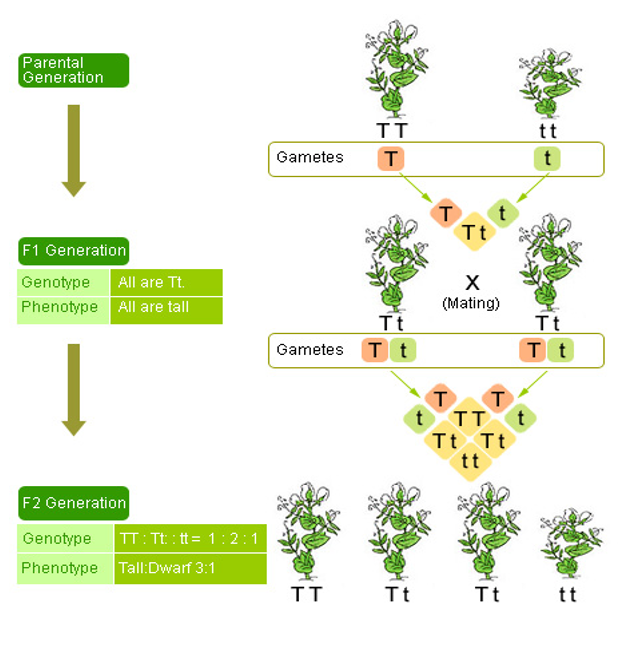Reciprocal Cross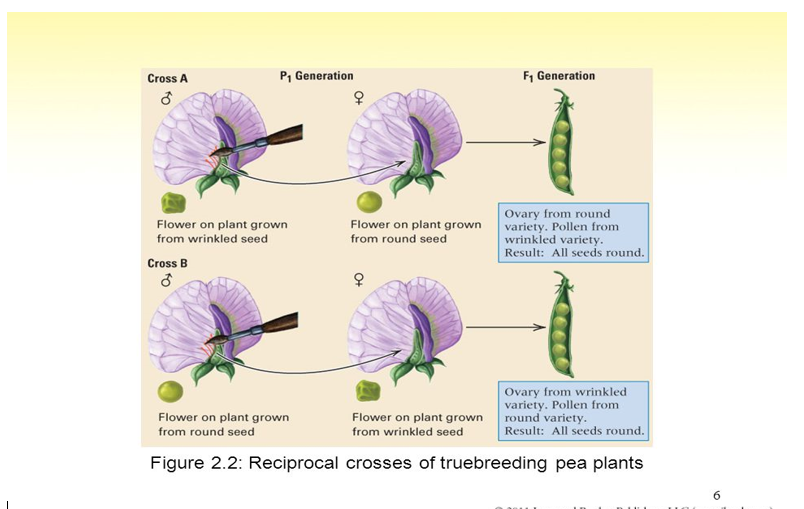Traits Present in the Progeny of the Hybrids

Mendel was the first to show that the characteristics of the progeny produced by a cross do not depend on which parent is the male and which the female. In this example, the seeds of the hybrid offspring are round whether the egg came from the round variety and the pollen from

the wrinkled variety (A) or the other way around (B).

The trait expressed in the hybrids Mendel called the dominant trait; the trait not expressed in the

hybrids he called recessive.

The principal observations from the experiments were:

• The F1 hybrids express only the dominant trait.
• In the F2 generation, plants with either the dominant or the recessive trait are present.
• In the F2 generation, there are approximately three times as many plants with the dominant trait as plants with the recessive trait. In other words, the F2 ratio of dominant : recessive equals approximately 3 : 1.

Mendel’s Genetic Hypothesis and Its Experimental Tests

Two  members of a gene pair (alleles) segregate (separate) from each other in the formation of gametes. Half of the gametes carry one allele and the other half carry the other allele.

Principle of Segregation

Confirmation of Mendel’s First Law Hypothesis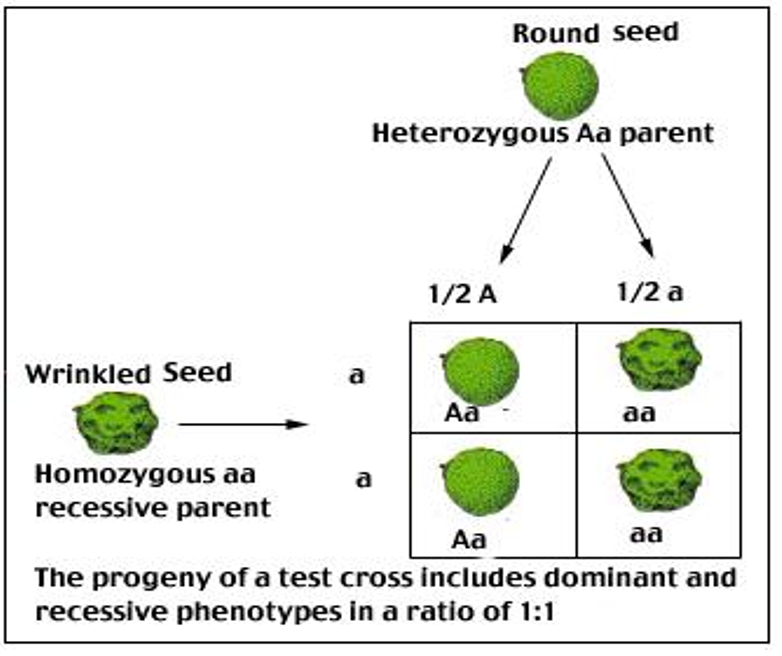True Breeding PlantA true-breeding plant is one that, when self-fertilized, only produces offspring with the same traits. The alleles for these type of plants are homozygous. Examples: The gene for seed shape in pea plants exists in two forms, one form or allele for round seed shape (R) and the other for wrinkled seed shape (r). A true-breeding round seed plant would be (RR) for that trait and a true-breeding wrinkled seed plant would be (rr).

Di-hybrid Cross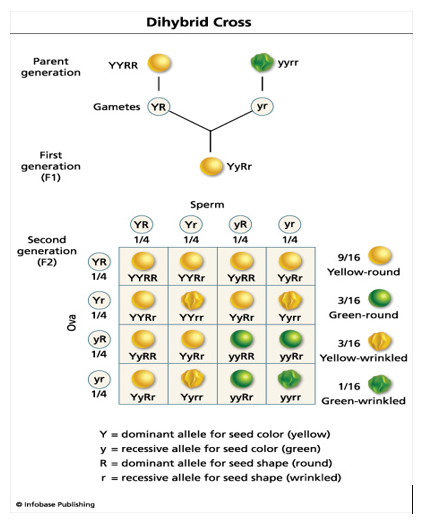• All seeds in the F1 had smooth yellow seeds. The F2 plants self-fertilized, and produced four phenotypes:
• 315 smooth yellow
• 108 smooth green
• 101 wrinkled yellow
• 32 wrinkled green

The purpose of the dihybrid cross was to determine if any relationship existed between different allelic pairs.

Meiosis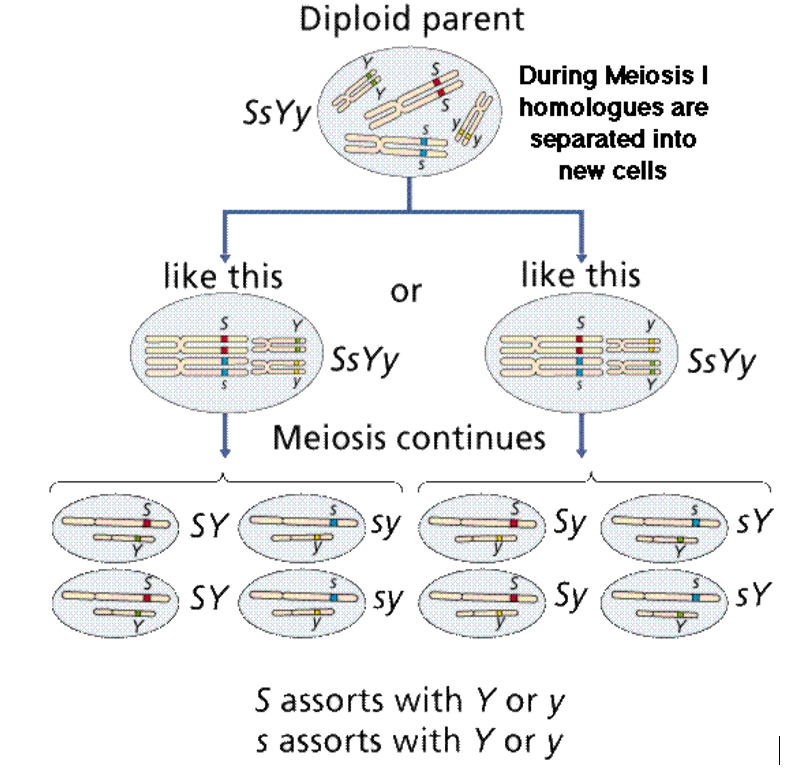The results of this experiment led Mendel to formulate his second law

A dihybrid cross tracks two traits. Both parents are heterozygous, and one allele for each trait exhibits complete dominance . This means that both parents have recessive alleles, but exhibit the dominant phenotype. The phenotype ratio predicted for dihybrid cross is 9:3:3:1.

The Principle of Independent Assortment:

Segregation of the members of any pair of alleles is independent of the segregation of other pairs in the formation of reproductive cells.

Or

Members of one gene pair segregate independently from other gene pairs during gamete formation

Confirmation of Mendel’s second Law Hypothesis

Mendel confirmed the results of his second law by performing a backcross – F1 dihybrid x recessive parent.

Let’s use the example of the yellow, round seeded F1.

The Backcross        YyRr     X        yyrr

⇓                   ⇓

Gametes           YR Yr  yR yr          yr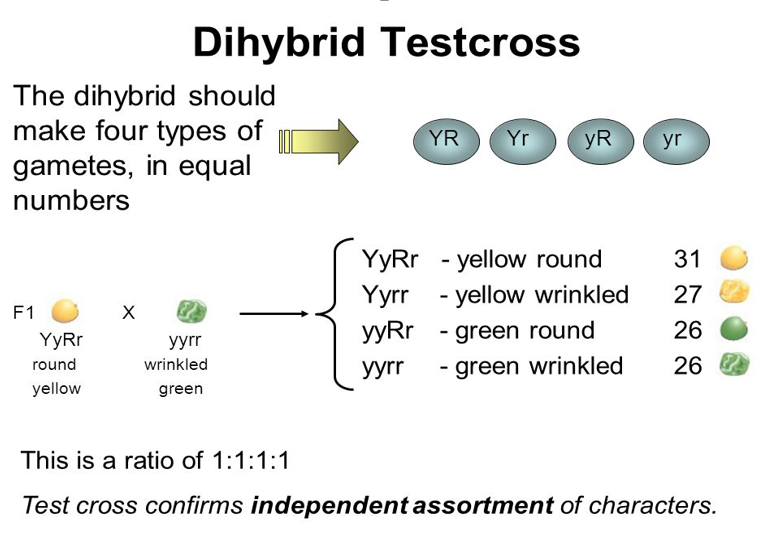The phenotypic ratio of the test cross is:

• 1 Yellow, Round Seed
• 1 Yellow, Wrinkled Seed
• 1 Green, Round Seed
• 1 Green, Wrinkled Seed

The ratio is 1:1:1:1

A Trihybrid Cross Example Using Mendel’s Sweet Peas

A trihybrid cross is between two individuals that are heterozygous for three different traits.

Our trihybrid cross example:

RrYyCc x RrYyCc is a trihybrid cross.

 The shape of the pea is controlled by one set of alleles, where round is completely dominant to wrinkled: RR = round Rr = round rr = wrinkled The second set of alleles in this example controls the color of the peas. Green is dominant to yellow: YY = green Yy = green yy = yellow

 The third set of alleles in this example controls the shape of the pea pod. Smooth  is completely dominant to constricted: CC = smooth Cc = smooth cc = constricted

The gametes for each parent in a trihybrid cross would be RYC, RYc, RyC, Ryc, rYC, rYc, ryC, ryc, with one-eighth of a chance for any of them.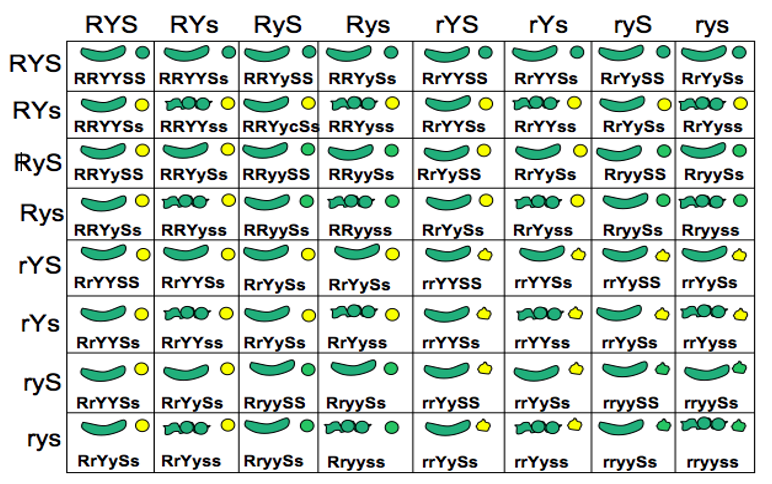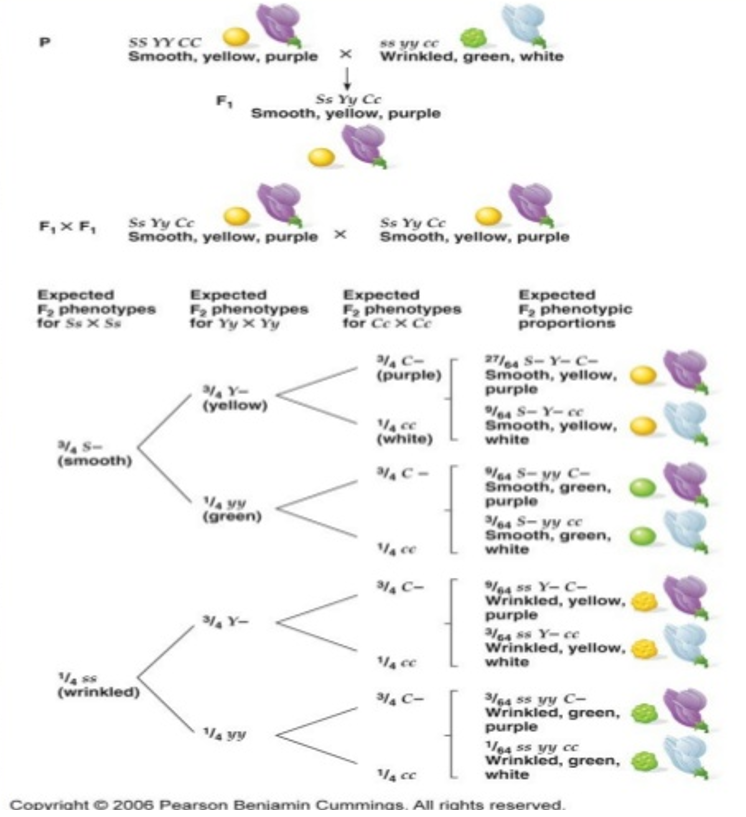27:9:9:9:3:3:3:1 ratio: a trihybrid cross yields a phenotypic ratio of 27:9:9:9:3:3:3:1. This reflects the phenotypes generated by the 64 genotypic combinations resulting from 8 different male gametes fertilizing 8 different female gametes.

Punnett square

A diagram that predicts the expected outcome of a genetic cross by considering all combinations of gametes in  the cross.

F1: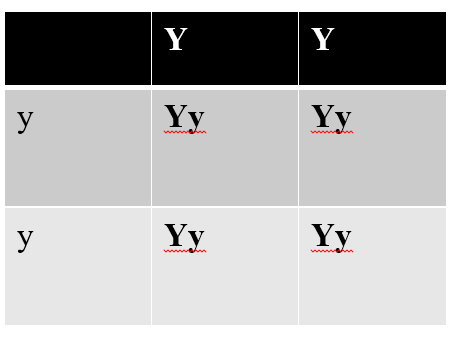F2: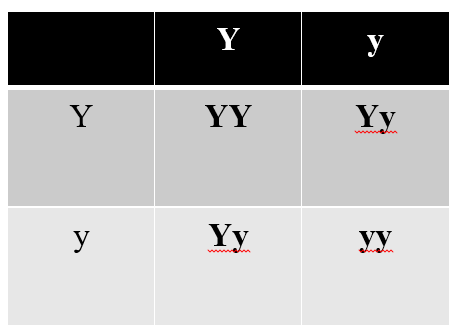Phenotypic ratio: 1:2:1 (3:1)

In case of dihybrid cross:

YYRR      X       yyrr          Parent generation

⇓

YR                      yr           Gametes

⇓

YyRr                       F1

Gamete Formation: Trihybrid cross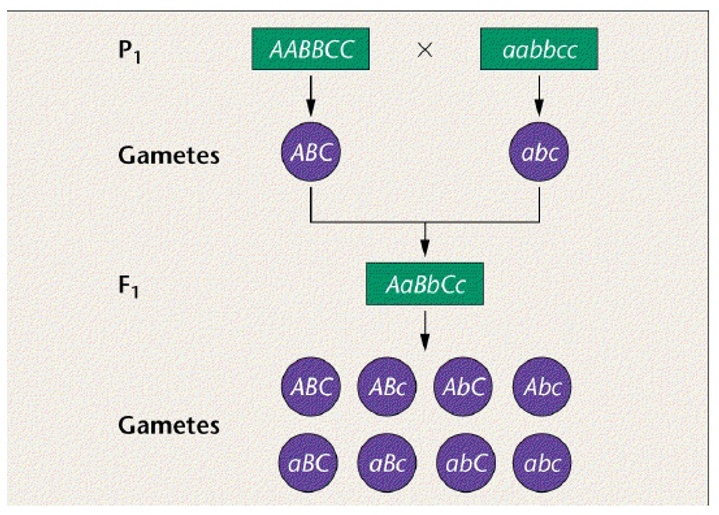What size of Punnett square needed for analysis?

Trihybrid Cross – Phenotypes  Forked-line Method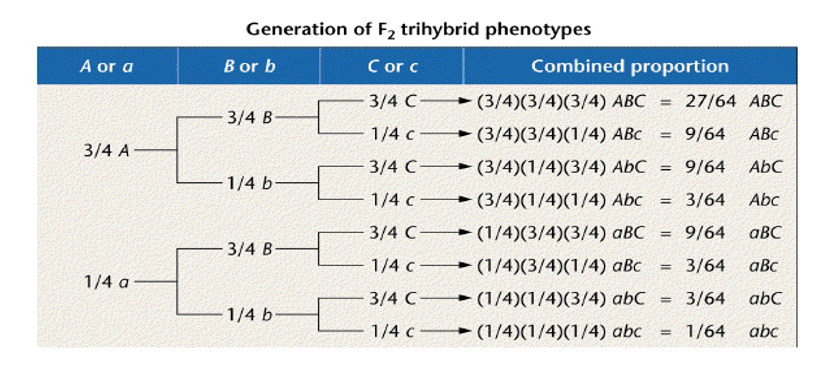Probability in Mendelian inheritance

A working knowledge of the rules of probability for predicting the outcome of chance events is basic to understanding the transmission of hereditary characteristics.

• Mendel’s laws of segregation and independent assortment reflect the same laws of probability
• The probability of each elementary outcome must be a non-negative number. The probability scale ranged from zero (an event with no chance of occurring) to one (an event that is certain to occur).
• The sum of the probabilities of all the elementary outcomes must equal to1.

Mendelian inheritance reflects rule of probability

• Mendel’s laws of segregation and independent assortment reflect the same laws of probability
• The probability of each elementary outcome must be a non-negative number. The probability scale ranged from zero (an event with no chance of occurring) to one (an event that is certain to occur).
• The sum of the probabilities of all the elementary outcomes must equal to 1.

Laws of probability help explain genetic events

Genetic ratios are most properly expressed as probabilities:

Ex. 3/4 tall: 1/4 dwarf

The probability of each zygote having the genetic potential for becoming tall is 3/4, etc.

How do we calculate the probability of 2 or more events happening at the same time?

Rule of multiplication (AND)

• Chance that 2 or more independent events will occur together
• When to use it:
• When you want to determine the probability that two or more independent events will occur together in some specific combination.
• How to use it:
• Compute the probability of each independent event.
• Then, multiply the individual probabilities to obtain the overall probability of these events occurring together.

Rule of Multiplication

Probability that 2 coins tossed at the same time will land heads up

Chance of tossing 1 with first coin = 1/2

Chance of tossing 1 with second coin = 1/2

Chance of rolling two 1’s = 1/2 X 1/2 = ¼

Similarly, the probability that a heterogyzous pea plant (Pp) will produce a white-flowered offspring (pp) depends on an ovum with a white allele mating with a sperm with a white allele.

This probability is 1/2 x 1/2 = 1/4.

The rule of multiplication also applies to dihybrid crosses.

• For a heterozygous parent (YyRr) the probability of producing a YR gamete is 1/2 x 1/2 = 1/4.
• We can use this to predict the probability of a particular F2 genotype without constructing a 16-part Punnett square.
• The probability that an F2 plant will have a YYRR genotype from a heterozygous parent is 1/16 (1/4 chance for a YR ovum and 1/4 chance for a YR sperm).

• The rule of addition also applies to genetic problems.
• Under the rule of addition, the probability of an event that can occur two or more different ways is the sum of the separate probabilities of those ways.
• probability of Bb x Bb ® Bb.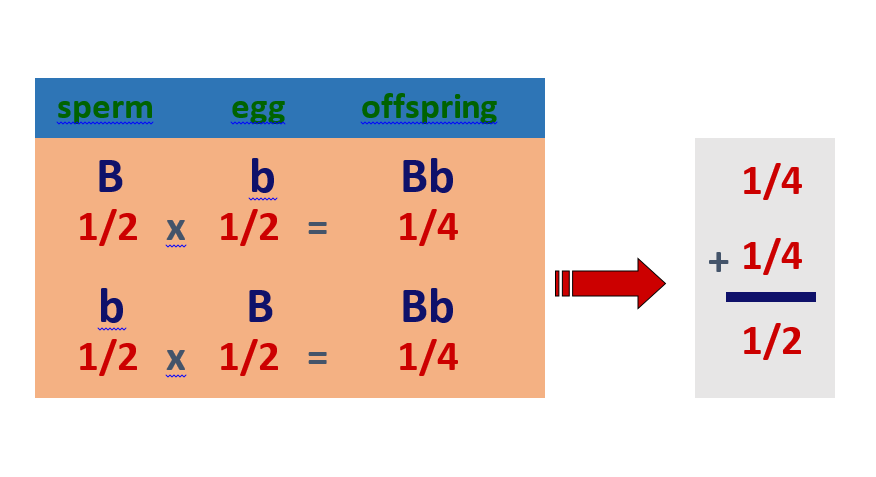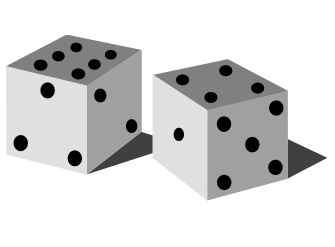• For outcomes that can occur more than one way (OR)
• What is the chance that you will roll either a 1 or a 6 with one die?
• Chance of rolling 1 = 1/6
• Chance of rolling 6 = 1/6
• Chance of rolling 1 or 6 = 1/6 + 1/6 = 2/6 = 1/3
• For example, there are two ways that F1 gametes can combine to form a heterozygote.
• The dominant allele could come from the sperm and the recessive from the ovum (probability = 1/4).
• Or, the dominant allele could come from the ovum and the recessive from the sperm (probability = 1/4).
• The probability of a heterozygote is 1/4 + 1/4 = 1/2.

The Chi-Square Test

An important question to answer in any genetic experiment is how can we decide if our data fits any of the Mendelian ratios we have discussed. A statistical test that can test out ratios is the Chi-Square or Goodness of Fit test.

Chi-Square Formula:Degrees of freedom (df) = n-1 where n is the number of classes

Let’s test the following data to determine if it fits a 9:3:3:1 ratio.Number of classes (n) = 4

df = n-1

= 4-1 = 3

Chi-square value = 0.47

By statistical convention, we use the 0.05 probability level as our critical value. If the calculated chi-square value is less than the 0 .05 value, we accept the hypothesis. If the value is greater than the value, we reject the hypothesis. Therefore, because the calculated chi-square value is greater than the we accept the hypothesis that the data fits a 9:3:3:1 ratio.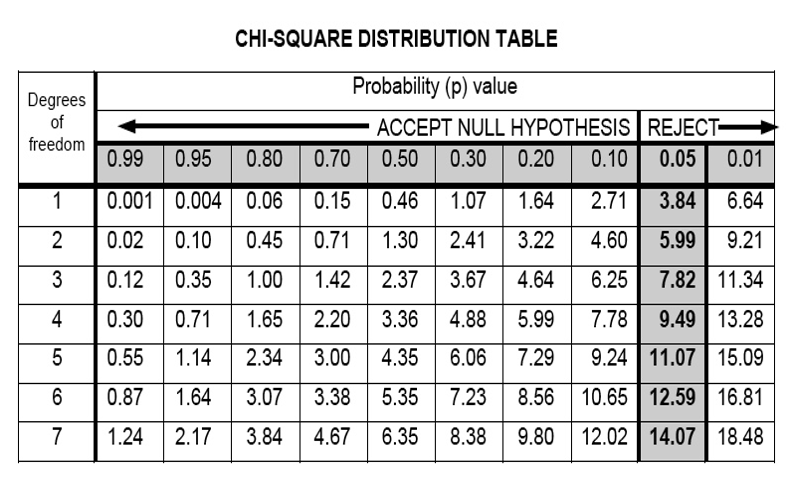We have finished our first topic of 2nd year Fundamental Genetics syllabus. Stay with us for the next topics.

5 1 vote
Article Rating## Mutagenic Agents: Types, Mechanism & Irradiation Breeding

Mutation breeding or mutagenesis is one of many great inventions of the 20th century. Until …

Subscribe
Notify of1 Comment
Inline Feedbacks